# 什么是可微编程`function damping(x, max) {\n  let y = Math.abs(x);\n  // 下面的参数都是来源于公式用数值拟合的结果\n  y = 0.82231 * max / (1 + 4338.47 / Math.pow(y, 1.14791));\n  return Math.round(x < 0 ? -y : y);\n}`## 可微编程和自动微分的关系y = 0.82231 * max / (1 + 4338.47 / Math.pow(y, 1.14791));`julia> guess = PointLight(Vec3(1.0), 20000.0, Vec3(1.0, 2.0, -7.0))\njulia> function loss_function(light)\n           rendered_color = raytrace(origin, direction, scene, light, eye_pos)\n           rendered_img = process_image(rendered_color, screen_size.w,\n                                        screen_size.h)\n           return mean((rendered_img .- reference_img).^2)\n       end\njulia> gs = gradient(x -> loss_function(x, image), guess)`### 可微编程实现智能应用程序

`enum PodcastCategory {\n    case comedy\n    case news\n    ...\n}\n\nenum PodcastSection {\n    case advertisement\n    case introduction\n    case body\n    case conclusion\n}\n\nstruct PodcastState {\n    let category: PodcastCategory\n    let section: PodcastSection\n}\n\nstruct PodcastSpeedModel {\n    var minSpeed, maxSpeed: Float\n    var categoryMultipliers: [PodcastCategory: Float]\n    var sectionMultipliers: [PodcastSection: Float]\n\n    /// Returns a podcast speed multiplier prediction for the given podcast category\n    /// and section.\n    func prediction(for state: PodcastState) -> Float {\n        let speed = categoryMultipliers[state.category] * sectionMultipliers[state.section]\n        if speed < minSpeed { return minSpeed }\n        if speed > maxSpeed { return maxSpeed }\n        return speed\n    }\n}`

1. 让用户手动设置速度，并在用户改变速度时记录观察结果的参数值。
2. 在收集到足够的观察值后，搜索参数值，使模型预测的速度接近用户的首选速度。如果找到这样的值，播放器会产生预测并自动设置速度。

“梯度下降”是执行这种搜索的算法，而支持可微编程的语言可以很容易地实现梯度下降。这是一些说明梯度下降的伪代码：

`// 首先，我们需要一个梯度下降的目标函数来最小化，这里使用平均绝对误差：\nstruct Observation {\n    var podcastState: PodcastState\n    var userSpeed: Float\n}\n\nfunc meanError(for model: PodcastSpeedModel, _ observations: [Observation]) -> Float {\n    var error: Float = 0\n    for observation in observations {\n        error += abs(model.prediction(for: observation.podcastState) - observation.userSpeed)\n    }\n    return error / Float(observations.count)\n}\n// 接下来，我们实现梯度下降算法。\nvar model = PodcastModel()\nlet observations = storage.observations()\nfor _ in 0..<1000 {\n    // The language differentiates `meanError` to get a "gradient", which is a value indicating\n    // how to change `model` in order to decrease the value of `meanError`.\n    let gradient = gradient(at: model) { meanError(for: \$0, observations) }\n\n    // Change `model` in the direction that decreased the value of `meanError`.\n    model -= 0.01 * gradient\n}`

# 用工具方程拟合`train_x = (0,500,1000,1500,2500,6000,8000,10000,12000)\ntrain_y = (0,90,160,210,260,347.5,357.5,367.5,377.5)`# 多项式拟合`\n# 安装 MiniConda 创建环境：并更新：pip install -U pip\nconda install -c apple tensorflow-deps\npip uninstall tensorflow-macos\npip uninstall tensorflow-metal\n# 进入工作目录启动 Jupyter Notebook 进行实验\njupyter notebook`

`import tensorflow as tf\n\n# Resets notebook state\ntf.keras.backend.clear_session()\n\nprint("Version: ", tf.__version__)\nprint("Eager mode: ", tf.executing_eagerly())\nprint(\n    "GPU is",\n    "available" if tf.config.list_physical_devices("GPU") else "NOT AVAILABLE")`

`Version:  2.8.0\nEager mode:  True\nGPU is available`

`train_x = [0,500,1000,1500,2500,6000,8000,10000,12000]\ntrain_y = [0,90,160,210,260,347.5,357.5,367.5,377.5]`

`\noptimizer = tf.keras.optimizers.Adam(0.1)\nt_x = tf.constant(train_x,dtype=tf.float32)\nt_y = tf.constant(train_y,dtype=tf.float32)\n\nwa = tf.Variable(0.,dtype = tf.float32,name='wa')\nwb = tf.Variable(0.,dtype = tf.float32,name='wb')\nwc = tf.Variable(0.,dtype = tf.float32,name='wc')\nwd = tf.Variable(0.,dtype = tf.float32,name='wd')\nvariables = [wa,wb,wc,wd]\nfor e in range(num):\n    with tf.GradientTape() as tape:\n        #预测函数\n        y_pred = tf.multiply(wa,t_x)+tf.multiply(w2,tf.pow(t_x,2))+tf.multiply(wb,tf.pow(t_x,2))+tf.multiply(wc,tf.pow(t_x,2))+wd\n        #损失函数\n        loss=tf.reduce_sum(tf.math.pow(y_pred-t_y,2))\n        #计算梯度\n        grads=tape.gradient(loss, variables)\n        #更新参数\n        optimizer.apply_gradients(grads_and_vars=zip(grads,variables))\n        if e % 100 == 0:\n            print("step: %i, loss: %f, a:%f, b:%f, c:%f, d:%f" % (e,loss,wa.numpy(),wb.numpy(),wc.numpy(),wd.numpy()))`

`step: 0, loss: 4.712249, a:0.100003, b:0.100003, c:0.100003, d:0.100003\nstep: 100, loss: 0.164529, a:1.204850, b:-0.219918, c:-0.219918, d:0.294863\nstep: 200, loss: 0.082497, a:1.994068, b:-0.615929, c:-0.615929, d:0.209093\nstep: 300, loss: 0.073271, a:2.291683, b:-0.766129, c:-0.766129, d:0.176420\n...\nstep: 9700, loss: 0.072893, a:2.371203, b:-0.804242, c:-0.804242, d:0.169179\nstep: 9800, loss: 0.072850, a:2.369858, b:-0.805587, c:-0.805587, d:0.167835\nstep: 9900, loss: 0.072853, a:2.369503, b:-0.805943, c:-0.805943, d:0.167479`

`plt.scatter(t_x,t_y,c='r')\ny_predict = tf.multiply(wa,t_x)+tf.multiply(w2,tf.pow(t_x,2))+tf.multiply(wb,tf.pow(t_x,2))+tf.multiply(wc,tf.pow(t_x,2))+wd\nprint(y_predict)\nplt.plot(t_x,y_predict,c='b')\nplt.show()\n# 输出：\ntf.Tensor(\n[0.16805027 0.2640069  0.3543707  0.4391417  0.59190494 0.95040166\n 1.0322142  1.0245409  0.92738193], shape=(9,), dtype=float32)`

`print(*(y_predict.numpy())*377.5)\n# 输出：\n63.43898 99.66261 133.77495 165.77599 223.4441 358.77664 389.66086 386.7642 350.08667\n# 真实数据：\ntrain_y = [0,90,160,210,260,347.5,357.5,367.5,377.5]`# scipy用curve_fit拟合4PL`from scipy import stats\nimport scipy.optimize as optimization\n\nxdata = t_x\nydata = t_y\n\ndef fourPL(x, A, B, C, D):\n    return ((A-D)/(1.0+((x/C)**(B))) + D)\n\nguess = [0, -0.3, 0.7, 1]\nparams, params_covariance = optimization.curve_fit(fourPL, xdata, ydata, guess)#, maxfev=1)\n\nx_min, x_max = np.amin(xdata), np.amax(xdata)\nxs = np.linspace(x_min, x_max, 1000)\nplt.scatter(xdata, ydata)\nplt.plot(xs, fourPL(xs, *params))\nplt.show()``print(*params)\n# 输出：# 使用工具得到的参数：\nA = 410.517236432893        A = 405.250160538176\nB = 1.1531891657236022      B = -1.17885294211307\nC = 1481.6957597831604      C = 1414.70383142617\nD = -0.16796047781164916    D = -0.516583385175963`y = 0.821 * max / (1 + 4527.779 / Math.pow(y, 1.153));

y = 0.82231 * max / (1 + 4338.47 / Math.pow(y, 1.14791));

<script src="https://cdn.jsdelivr.net/npm/vue"></script>

<script src="http://static.runoob.com/assets/vue/1.0.11/vue.min.js"></script>即可。

# 神经网络的拟合

`model = tf.keras.Sequential() \n# 添加层\n# 注：input_dim(输入神经元个数)只需要在输入层重视设置，后面的网络可以自动推断出该层的对应输入\nmodel.add(tf.keras.layers.Dense(units=10, input_dim=1, activation='selu'))\nmodel.add(tf.keras.layers.Dense(units=10, input_dim=1, activation='selu'))\nmodel.add(tf.keras.layers.Dense(units=10, input_dim=1, activation='selu'))\nmodel.add(tf.keras.layers.Dense(units=10, input_dim=1, activation='selu'))\nmodel.add(tf.keras.layers.Dense(units=10, input_dim=1, activation='selu'))\nmodel.add(tf.keras.layers.Dense(units=10, input_dim=1, activation='selu'))\nmodel.add(tf.keras.layers.Dense(units=10, input_dim=1, activation='selu'))\nmodel.add(tf.keras.layers.Dense(units=10, input_dim=1, activation='selu'))\nmodel.add(tf.keras.layers.Dense(units=10, input_dim=1, activation='selu'))\nmodel.add(tf.keras.layers.Dense(units=10, input_dim=1, activation='selu'))\n#                                   神经元个数  输入神经元个数 激活函数\nmodel.add(tf.keras.layers.Dense(units=1, activation='selu'))\n#                               输出神经元个数 \n# 2 设置优化器和损失函数\nmodel.compile(optimizer='adam', loss='mse')\n#                 优化器         损失函数(均方误差) \n# 3 训练\nhistory = model.fit(t_x, t_y,epochs=2000) \n# 4 预测\ny_pred = model.predict(t_x)`

`1/1 [==============================] - 1s 573ms/step - loss: 74593.5156\nEpoch 2/2000\n1/1 [==============================] - 0s 38ms/step - loss: 74222.5469\nEpoch 3/2000\n1/1 [==============================] - 0s 31ms/step - loss: 74039.7734\n...\nEpoch 1994/2000\n1/1 [==============================] - 0s 26ms/step - loss: 0.3370\nEpoch 1995/2000\n1/1 [==============================] - 0s 25ms/step - loss: 0.3370\nEpoch 1996/2000\n1/1 [==============================] - 0s 24ms/step - loss: 0.3370\nEpoch 1997/2000\n1/1 [==============================] - 0s 26ms/step - loss: 0.3370\nEpoch 1998/2000\n1/1 [==============================] - 0s 24ms/step - loss: 0.3370\nEpoch 1999/2000\n1/1 [==============================] - 0s 25ms/step - loss: 0.3369\nEpoch 2000/2000\n1/1 [==============================] - 0s 24ms/step - loss: 0.3369`

`# 5 画图\nplt.scatter(xdata, ydata)\nplt.plot(t_x, y_pred, 'r-', lw=5)\nplt.show()``print(t_x.reshape(-1, 1))     print(model.predict(t_x.reshape(-1, 1)))\n# 输出：预测输出：\n[[    0.]\n [  500.]\n [ 1000.]\n [ 1500.]\n [ 2500.]\n [ 6000.]\n [ 8000.]\n [10000.]\n [12000.]]\nprint(t_y.reshape(-1,1))\n# 输出：\n[[  0. ]                    [-1.7345996]\n [ 90. ]                    [90.01992]\n [160. ]                    [159.91837]\n [210. ]                    [210.06012]\n [260. ]                    [260.01797] \n [347.5]                    [347.43182] \n [357.5]                    [357.57867] \n [367.5]                    [367.53287] \n [377.5]]                   [377.4857]`

# 浏览器执行示例

`model.save('saved_model/w4model')`

`tensorflowjs_converter --input_format=tf_saved_model \\\n--output_node_names="w4model" \\\n--saved_model_tags=serve ./saved_model/w4model ./web_model`

`"dependencies": {\n    "@tensorflow/tfjs": "^3.18.0",\n    "@tensorflow/tfjs-converter": "^3.18.0"\n  },`

`import * as tf from "@tensorflow/tfjs";\nimport { loadGraphModel } from "@tensorflow/tfjs-converter";`

`import * as tf from "@tensorflow/tfjs";\nimport { loadGraphModel } from "@tensorflow/tfjs-converter";\nwindow.onload = async () => {\n  const resultElement = document.getElementById("result");\n  const MODEL_URL = "model.json";\n\n  console.time("Loading of model");\n  const model = await loadGraphModel(MODEL_URL);\n  console.timeEnd("Loading of model");\n\n  const test_data = tf.tensor([\n    [0.0],\n    [500.0],\n    [1000.0],\n    [1500.0],\n    [2500.0],\n    [6000.0],\n    [8000.0],\n    [10000.0],\n    [12000.0],\n  ]);\n  tf.print(test_data);\n  console.time("Loading of model");\n  let outputs = model.execute(test_data);\n  console.timeEnd("execute：");\n  tf.print(outputs);\n  resultElement.innerText = outputs.toString();\n};`

`[Violation] 'load' handler took 340ms\nindex.js:12 Loading of model: 67.19482421875 ms\nprint.ts:34 Tensor\n    [[0    ],\n     [500  ],\n     [1000 ],\n     [1500 ],\n     [2500 ],\n     [6000 ],\n     [8000 ],\n     ,\n     ]\nindex.js:28 execute: 257.47607421875 ms\nprint.ts:34 Tensor\n    [[-1.7345995 ],\n     [90.0198822 ],\n     [159.9183655],\n     [210.0600586],\n     [260.0179443],\n     [347.4320068],\n     [357.5788269],\n     [367.5332947],\n     [377.4856262]]`

`import * as tf from "@tensorflow/tfjs";\nimport { loadGraphModel } from "@tensorflow/tfjs-converter";\nwindow.onload = async () => {\n  // 新加入 webgl 硬件加速能力\n  tf.setBackend("webgl");\n  console.log(tf.getBackend());\n  // 打印当前后端信息\n  const resultElement = document.getElementById("result");\n  const MODEL_URL = "model.json";`

`damping(x, max) {\n            let y = Math.abs(x);\n            y = 0.821 * max / (1 + 4527.779 / Math.pow(y, 1.153));\n            return Math.round(x < 0 ? -y : y);\n          },`

","body_lake":"

# 什么是可微编程

## 可微编程和自动微分的关系

y = 0.82231 * max / (1 + 4338.47 / Math.pow(y, 1.14791));

### 可微编程实现智能应用程序

1. 让用户手动设置速度，并在用户改变速度时记录观察结果的参数值。
2. 在收集到足够的观察值后，搜索参数值，使模型预测的速度接近用户的首选速度。如果找到这样的值，播放器会产生预测并自动设置速度。

“梯度下降”是执行这种搜索的算法，而支持可微编程的语言可以很容易地实现梯度下降。这是一些说明梯度下降的伪代码：

# scipy用curve_fit拟合4PL

y = 0.821 * max / (1 + 4527.779 / Math.pow(y, 1.153));

y = 0.82231 * max / (1 + 4338.47 / Math.pow(y, 1.14791));

<script src="https://cdn.jsdelivr.net/npm/vue"></script>

<script src="http://static.runoob.com/assets/vue/1.0.11/vue.min.js"></script>即可。

# 浏览器执行示例# 什么是可微编程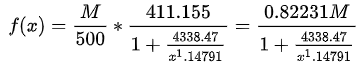```function damping(x, max) {
let y = Math.abs(x);
// 下面的参数都是来源于公式用数值拟合的结果
y = 0.82231 * max / (1 + 4338.47 / Math.pow(y, 1.14791));
return Math.round(x < 0 ? -y : y);
}```## 可微编程和自动微分的关系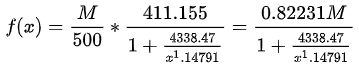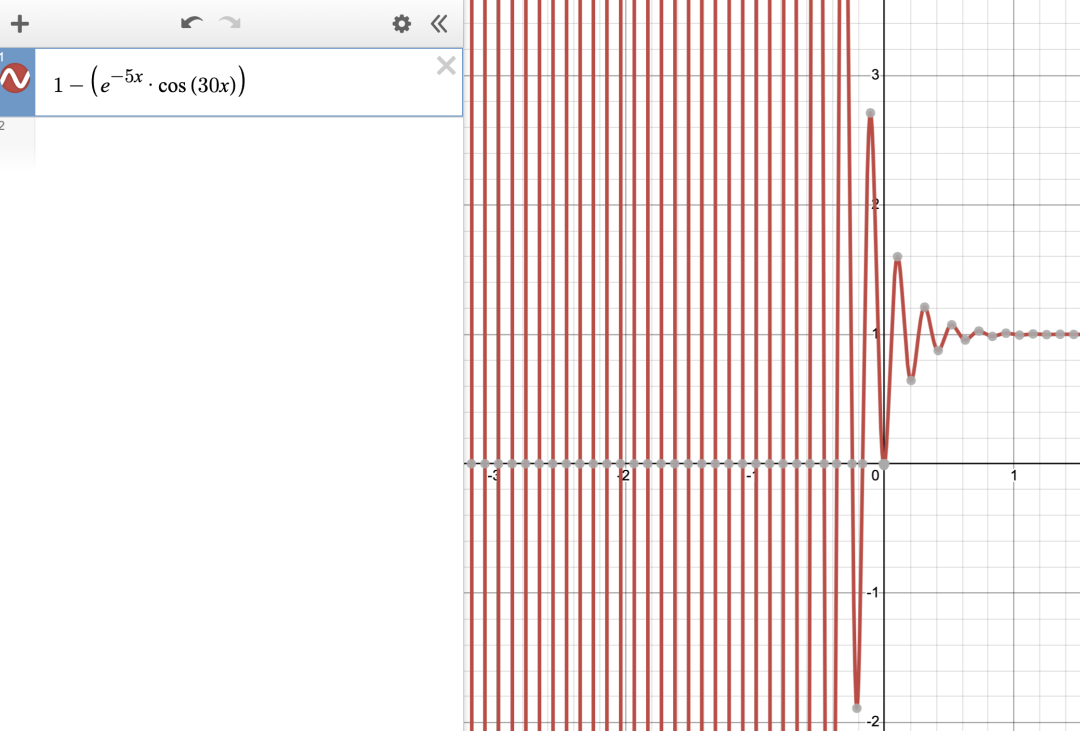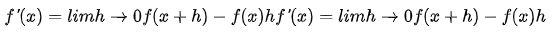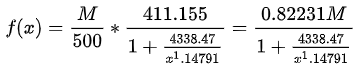y = 0.82231 * max / (1 + 4338.47 / Math.pow(y, 1.14791));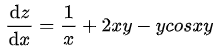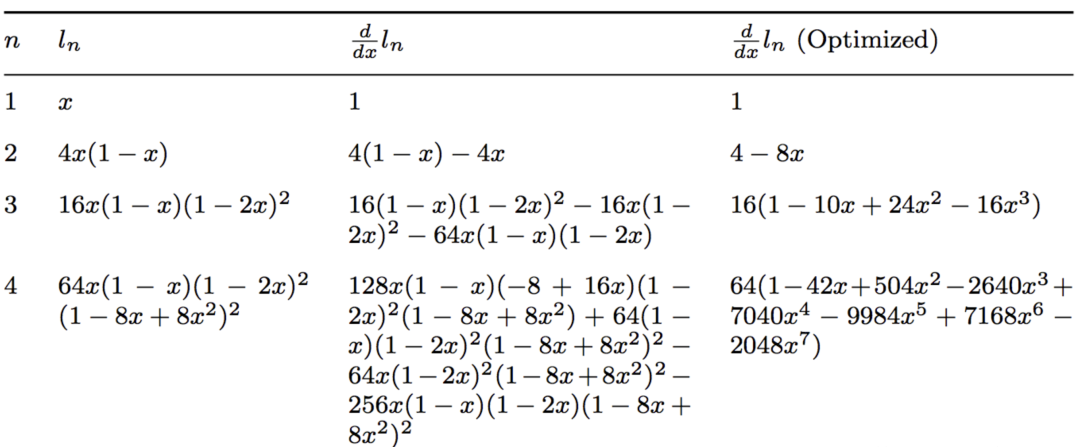```julia> guess = PointLight(Vec3(1.0), 20000.0, Vec3(1.0, 2.0, -7.0))
julia> function loss_function(light)
rendered_color = raytrace(origin, direction, scene, light, eye_pos)
rendered_img = process_image(rendered_color, screen_size.w,
screen_size.h)
return mean((rendered_img .- reference_img).^2)
end
julia> gs = gradient(x -> loss_function(x, image), guess)```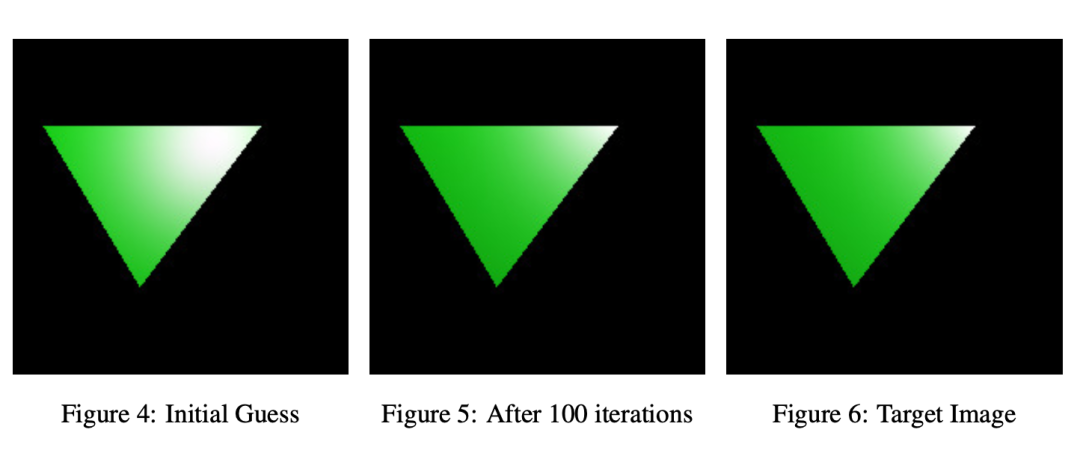### 可微编程实现智能应用程序

```enum PodcastCategory {
case comedy
case news
...
}

enum PodcastSection {
case introduction
case body
case conclusion
}

struct PodcastState {
let category: PodcastCategory
let section: PodcastSection
}

struct PodcastSpeedModel {
var minSpeed, maxSpeed: Float
var categoryMultipliers: [PodcastCategory: Float]
var sectionMultipliers: [PodcastSection: Float]

/// Returns a podcast speed multiplier prediction for the given podcast category
/// and section.
func prediction(for state: PodcastState) -> Float {
let speed = categoryMultipliers[state.category] * sectionMultipliers[state.section]
if speed < minSpeed { return minSpeed }
if speed > maxSpeed { return maxSpeed }
return speed
}
}```

1. 让用户手动设置速度，并在用户改变速度时记录观察结果的参数值。
2. 在收集到足够的观察值后，搜索参数值，使模型预测的速度接近用户的首选速度。如果找到这样的值，播放器会产生预测并自动设置速度。

“梯度下降”是执行这种搜索的算法，而支持可微编程的语言可以很容易地实现梯度下降。这是一些说明梯度下降的伪代码：

```// 首先，我们需要一个梯度下降的目标函数来最小化，这里使用平均绝对误差：
struct Observation {
var podcastState: PodcastState
var userSpeed: Float
}

func meanError(for model: PodcastSpeedModel, _ observations: [Observation]) -> Float {
var error: Float = 0
for observation in observations {
error += abs(model.prediction(for: observation.podcastState) - observation.userSpeed)
}
return error / Float(observations.count)
}
// 接下来，我们实现梯度下降算法。
var model = PodcastModel()
let observations = storage.observations()
for _ in 0..<1000 {
// The language differentiates `meanError` to get a "gradient", which is a value indicating
// how to change `model` in order to decrease the value of `meanError`.

// Change `model` in the direction that decreased the value of `meanError`.
}```

# 用工具方程拟合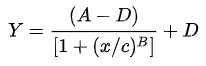```train_x = (0,500,1000,1500,2500,6000,8000,10000,12000)
train_y = (0,90,160,210,260,347.5,357.5,367.5,377.5)```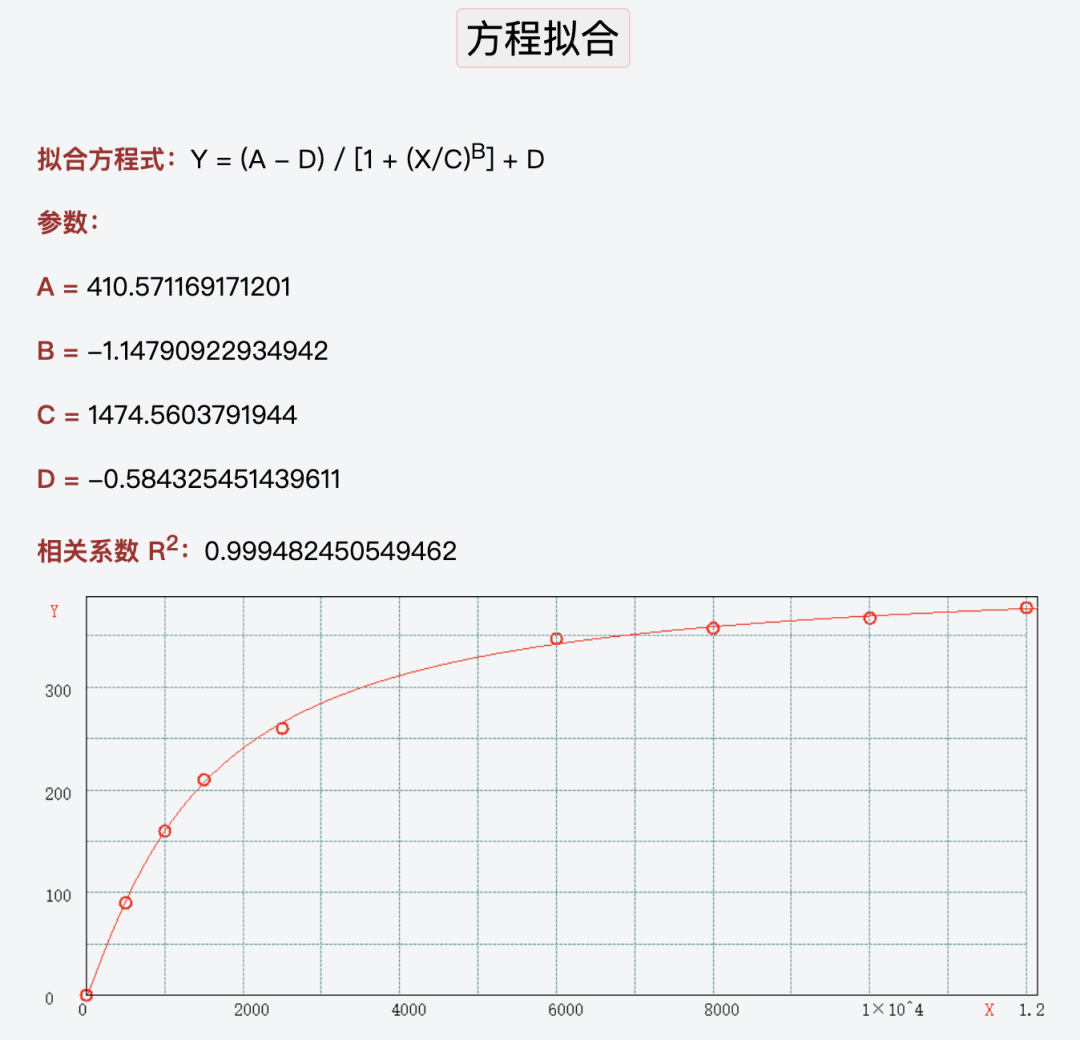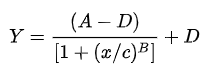# 多项式拟合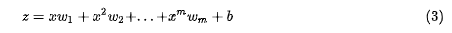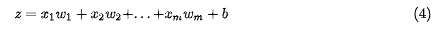```# 安装 MiniConda 创建环境：并更新：pip install -U pip
conda install -c apple tensorflow-deps
pip uninstall tensorflow-macos
pip uninstall tensorflow-metal
# 进入工作目录启动 Jupyter Notebook 进行实验
jupyter notebook```

```import tensorflow as tf

# Resets notebook state
tf.keras.backend.clear_session()

print("Version: ", tf.__version__)
print("Eager mode: ", tf.executing_eagerly())
print(
"GPU is",
"available" if tf.config.list_physical_devices("GPU") else "NOT AVAILABLE")```

```Version:  2.8.0
Eager mode:  True
GPU is available```

```train_x = [0,500,1000,1500,2500,6000,8000,10000,12000]
train_y = [0,90,160,210,260,347.5,357.5,367.5,377.5]```

```optimizer = tf.keras.optimizers.Adam(0.1)
t_x = tf.constant(train_x,dtype=tf.float32)
t_y = tf.constant(train_y,dtype=tf.float32)

wa = tf.Variable(0.,dtype = tf.float32,name='wa')
wb = tf.Variable(0.,dtype = tf.float32,name='wb')
wc = tf.Variable(0.,dtype = tf.float32,name='wc')
wd = tf.Variable(0.,dtype = tf.float32,name='wd')
variables = [wa,wb,wc,wd]
for e in range(num):
#预测函数
y_pred = tf.multiply(wa,t_x)+tf.multiply(w2,tf.pow(t_x,2))+tf.multiply(wb,tf.pow(t_x,2))+tf.multiply(wc,tf.pow(t_x,2))+wd
#损失函数
loss=tf.reduce_sum(tf.math.pow(y_pred-t_y,2))
#计算梯度
#更新参数
if e % 100 == 0:
print("step: %i, loss: %f, a:%f, b:%f, c:%f, d:%f" % (e,loss,wa.numpy(),wb.numpy(),wc.numpy(),wd.numpy()))```

```step: 0, loss: 4.712249, a:0.100003, b:0.100003, c:0.100003, d:0.100003
step: 100, loss: 0.164529, a:1.204850, b:-0.219918, c:-0.219918, d:0.294863
step: 200, loss: 0.082497, a:1.994068, b:-0.615929, c:-0.615929, d:0.209093
step: 300, loss: 0.073271, a:2.291683, b:-0.766129, c:-0.766129, d:0.176420
...
step: 9700, loss: 0.072893, a:2.371203, b:-0.804242, c:-0.804242, d:0.169179
step: 9800, loss: 0.072850, a:2.369858, b:-0.805587, c:-0.805587, d:0.167835
step: 9900, loss: 0.072853, a:2.369503, b:-0.805943, c:-0.805943, d:0.167479```

```plt.scatter(t_x,t_y,c='r')
y_predict = tf.multiply(wa,t_x)+tf.multiply(w2,tf.pow(t_x,2))+tf.multiply(wb,tf.pow(t_x,2))+tf.multiply(wc,tf.pow(t_x,2))+wd
print(y_predict)
plt.plot(t_x,y_predict,c='b')
plt.show()
# 输出：
tf.Tensor(
[0.16805027 0.2640069  0.3543707  0.4391417  0.59190494 0.95040166
1.0322142  1.0245409  0.92738193], shape=(9,), dtype=float32)```

```print(*(y_predict.numpy())*377.5)
# 输出：
63.43898 99.66261 133.77495 165.77599 223.4441 358.77664 389.66086 386.7642 350.08667
# 真实数据：
train_y = [0,90,160,210,260,347.5,357.5,367.5,377.5]```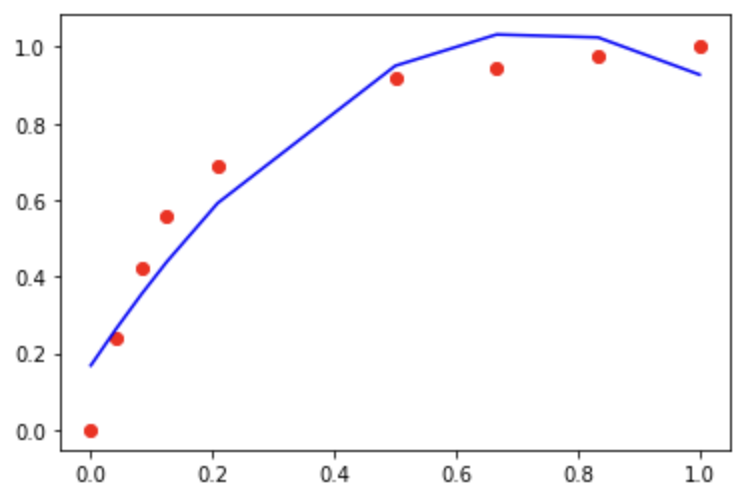# scipy用curve_fit拟合4PL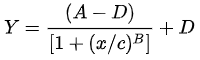```from scipy import stats
import scipy.optimize as optimization

xdata = t_x
ydata = t_y

def fourPL(x, A, B, C, D):
return ((A-D)/(1.0+((x/C)**(B))) + D)

guess = [0, -0.3, 0.7, 1]
params, params_covariance = optimization.curve_fit(fourPL, xdata, ydata, guess)#, maxfev=1)

x_min, x_max = np.amin(xdata), np.amax(xdata)
xs = np.linspace(x_min, x_max, 1000)
plt.scatter(xdata, ydata)
plt.plot(xs, fourPL(xs, *params))
plt.show()```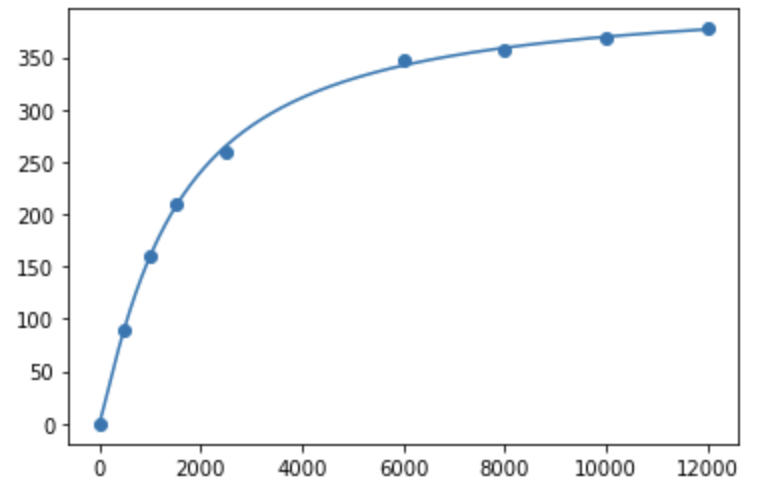```print(*params)
# 输出：# 使用工具得到的参数：
A = 410.517236432893        A = 405.250160538176
B = 1.1531891657236022      B = -1.17885294211307
C = 1481.6957597831604      C = 1414.70383142617
D = -0.16796047781164916    D = -0.516583385175963```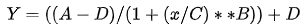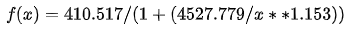y = 0.821 * max / (1 + 4527.779 / Math.pow(y, 1.153));

y = 0.82231 * max / (1 + 4338.47 / Math.pow(y, 1.14791));

<script src="https://cdn.jsdelivr.net/npm/vue"></script>

<script src="http://static.runoob.com/assets/vue/1.0.11/vue.min.js"></script>即可。

# 神经网络的拟合

```model = tf.keras.Sequential()
# 添加层
# 注：input_dim(输入神经元个数)只需要在输入层重视设置，后面的网络可以自动推断出该层的对应输入
#                                   神经元个数  输入神经元个数 激活函数
#                               输出神经元个数
# 2 设置优化器和损失函数
#                 优化器         损失函数(均方误差)
# 3 训练
history = model.fit(t_x, t_y,epochs=2000)
# 4 预测
y_pred = model.predict(t_x)```

```1/1 [==============================] - 1s 573ms/step - loss: 74593.5156
Epoch 2/2000
1/1 [==============================] - 0s 38ms/step - loss: 74222.5469
Epoch 3/2000
1/1 [==============================] - 0s 31ms/step - loss: 74039.7734
...
Epoch 1994/2000
1/1 [==============================] - 0s 26ms/step - loss: 0.3370
Epoch 1995/2000
1/1 [==============================] - 0s 25ms/step - loss: 0.3370
Epoch 1996/2000
1/1 [==============================] - 0s 24ms/step - loss: 0.3370
Epoch 1997/2000
1/1 [==============================] - 0s 26ms/step - loss: 0.3370
Epoch 1998/2000
1/1 [==============================] - 0s 24ms/step - loss: 0.3370
Epoch 1999/2000
1/1 [==============================] - 0s 25ms/step - loss: 0.3369
Epoch 2000/2000
1/1 [==============================] - 0s 24ms/step - loss: 0.3369```

```# 5 画图
plt.scatter(xdata, ydata)
plt.plot(t_x, y_pred, 'r-', lw=5)
plt.show()```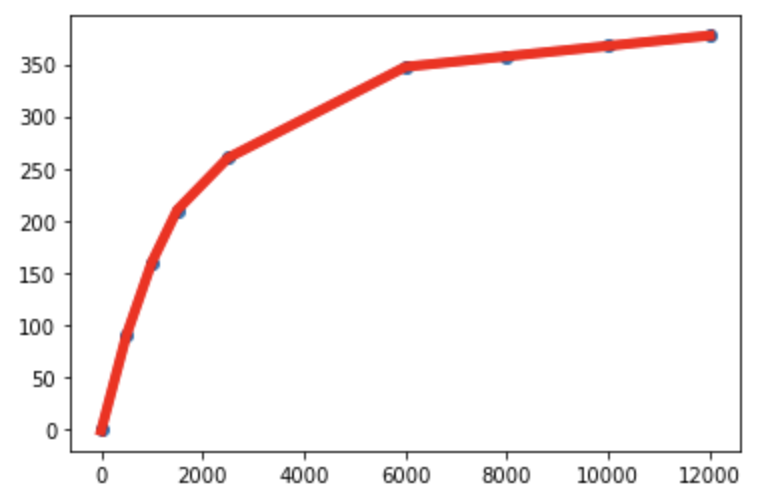```print(t_x.reshape(-1, 1))     print(model.predict(t_x.reshape(-1, 1)))
# 输出：预测输出：
[[    0.]
[  500.]
[ 1000.]
[ 1500.]
[ 2500.]
[ 6000.]
[ 8000.]
[10000.]
[12000.]]
print(t_y.reshape(-1,1))
# 输出：
[[  0. ]                    [-1.7345996]
[ 90. ]                    [90.01992]
[160. ]                    [159.91837]
[210. ]                    [210.06012]
[260. ]                    [260.01797]
[347.5]                    [347.43182]
[357.5]                    [357.57867]
[367.5]                    [367.53287]
[377.5]]                   [377.4857]```

# 浏览器执行示例

`model.save('saved_model/w4model')`

```tensorflowjs_converter --input_format=tf_saved_model \
--output_node_names="w4model" \
--saved_model_tags=serve ./saved_model/w4model ./web_model```

```"dependencies": {
"@tensorflow/tfjs": "^3.18.0",
"@tensorflow/tfjs-converter": "^3.18.0"
},```

```import * as tf from "@tensorflow/tfjs";
import { loadGraphModel } from "@tensorflow/tfjs-converter";```

```import * as tf from "@tensorflow/tfjs";
import { loadGraphModel } from "@tensorflow/tfjs-converter";
window.onload = async () => {
const resultElement = document.getElementById("result");
const MODEL_URL = "model.json";

const test_data = tf.tensor([
[0.0],
[500.0],
[1000.0],
[1500.0],
[2500.0],
[6000.0],
[8000.0],
[10000.0],
[12000.0],
]);
tf.print(test_data);
let outputs = model.execute(test_data);
console.timeEnd("execute：");
tf.print(outputs);
resultElement.innerText = outputs.toString();
};```

```[Violation] 'load' handler took 340ms
print.ts:34 Tensor
[[0    ],
[500  ],
[1000 ],
[1500 ],
[2500 ],
[6000 ],
[8000 ],
,
]
index.js:28 execute: 257.47607421875 ms
print.ts:34 Tensor
[[-1.7345995 ],
[90.0198822 ],
[159.9183655],
[210.0600586],
[260.0179443],
[347.4320068],
[357.5788269],
[367.5332947],
[377.4856262]]```

```import * as tf from "@tensorflow/tfjs";
import { loadGraphModel } from "@tensorflow/tfjs-converter";
window.onload = async () => {
// 新加入 webgl 硬件加速能力
tf.setBackend("webgl");
console.log(tf.getBackend());
// 打印当前后端信息
const resultElement = document.getElementById("result");
const MODEL_URL = "model.json";```

```damping(x, max) {
let y = Math.abs(x);
y = 0.821 * max / (1 + 4527.779 / Math.pow(y, 1.153));
return Math.round(x < 0 ? -y : y);
},```